Sunday, 29 November 2009

An unintuitive probability problem

Probability can do strange things to your mind. This week I had a probability problem where every time I tried to use intuition to solve it I ended up going completely wrong. I thought I'd share it as I think it's interesting.

Consider a one dimensional random walk. At each time step my walker will go left with probability, and right with probability. It stays where it is with probability. Furthermore these probabilities are dependent on the walker's position in space, so it's reallyand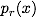. I'm imagining I'm on a finite line of length, L, although it doesn't matter too much.

Now if, then we just have a normal random walker. In my problem I have the following setup: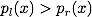but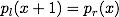. What does this mean? At any given point, x, my walker is more likely to go left than right. If it does go left it will come back with the same rate (although it's more likely to go left again).So here's the question: If I leave this for a really long time, what is the equilibrium probability distribution for the walkers position,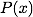?

At any given site the chances are you are more likely to go left than right. Therefore, this introduces a bias to go left. The walker is more likely to be on the left hand side of the line after a long time.

Because the transition rates are much faster on the left than the right, if the walker happens to go to the left it will much more quickly return. If the walker happens to go right then it's going to take a long time to return. Therefore you're more likely to find it on the right.

Well it's a little of both. The correct answer is that there is equal probability of finding the walker absolutely anywhere. The reason for this does not come from intuition. At equilibrium we have the condition that there must be no probability "current". That is to say that the probability of being found at a given site must not change in time. This can be expressed mathematically by the master equation (if you don't want to do the maths we'll meet back at the end):You have to think of the ps as rates for this to work. In our case we have that the rate for going left or right is the same as making the reverse move. The master equation then reduces toand the solution is that. So how does this sit with our intuitive ideas above? Well perhaps it's best to look at a trace of such a random walk. In the below example we have that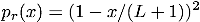. What happens is that the walker does indeed move quickly at small x and slowly at large x (as in intuitive answer 2), but the slowness at large x seems to reflect the walker away. When it does manage to penetrate the barrier it takes a long time to come back. The average behaviour is that every site, x, is occupied for the same amount of time.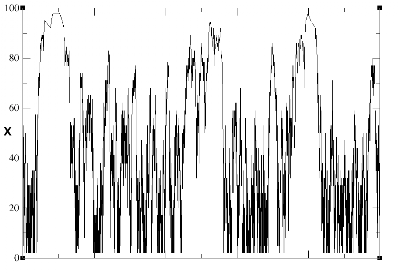So there it is. Never trust your instincts when it comes to probability, well not mine at least.

Oh, and on a separate note, putting maths into a blog post is a bloody nightmare - any ideas?
EDIT: Some maths fixed...

1.I provided another intuition (3?)

If we sit in the middle of x and x+1, we'll see the flux coming from the right (x+1) and flux coming from the left (x). In equilibrium they should be the same. So

[P(x)][p_r(x)] = [P(x+1)][p_l(x+1)].

Since p_r(x) = p_l(x+1). P(x) = P(x+1).

Quite ituitive, but I don't know if this make sense rigorously though. =)

2.Yes this is right. Your condition for equilibrium is slightly stronger than the one I wrote down, it's known as "detailed balance" and enforces a stationary distribution with no limit cycles.

For the chain as I've described it it's totally appropriate as I don't see what limit cycle we could have.

It was writing down something like this that eventually stopped me being confused. Whether or not it's intuitive I suppose is somewhat subjective!